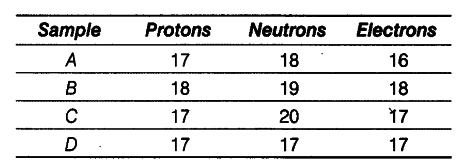# On the basis of the number of protons, neutrons and electrons in the samples given below identify

On the basis of the number of protons, neutrons and electrons in the samples given below identify
(i) the cation.
(ii) the pair of isobars, and
(iii) the pair of isotopes.(i) Sample A has more protons than the electrons.
Hence, it is a cation.
(ii) Sample B and C have same mass number (Mass number = Number of protons + number of
neutrons = 37) but different atomic numbers
(i.e. 18 and 17 respectively). Hence, they are a pair of isobars.
(iii) Samples C and D have same atomic number but different mass numbers. Hence, they are a pair of isotopes.